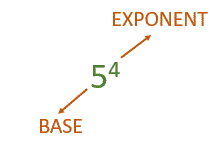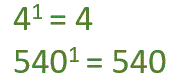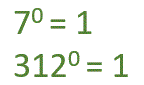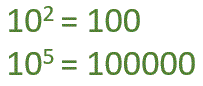Smartick is a fun way to learn math!Oct24

# Powers in Math

Hello! How did your week start off?

Are you ready to learn about what powers are today? Yes! You heard correctly, today we are going to focus on powers.

Do you know what powers are for? We use powers to simplify multiplication problems that use more than one of the same number. The power of a number says how many times to multiply the number by itself.

Let’s take a look at an example:

## 5 x 5 x 5 x 5

This expression can be written in a shorter way using something called exponents. We are multiplying the number 5 four times. To put this number in powers, first, we write the number 5 and above on the right side, we write a small 4.

This expression can be written in a shorter way using something called exponents:The number 5 is the base, which is the number that is multiplied and the number 4 is the exponent, which is the number of times the base is multiplied by.

So then, how do we verbally express this? “5 to the power of 4.“

There are some powers that are a bit special:

• Exponents equal to 1: Any number to the power of 1 will always be the same number.• Exponents equal to 0: Any number to the power of 0 will always be 1.• Base equal to 10: The exponent indicates the number of zeros that the answer will have.I hope this post helps you understand powers.

And if you want to learn much more math, register with Smartick for a free trial.

Fun is our brain’s favorite way of learning
Diane Ackerman
Smartick is a fun way to learn math
• 15 fun minutes a day